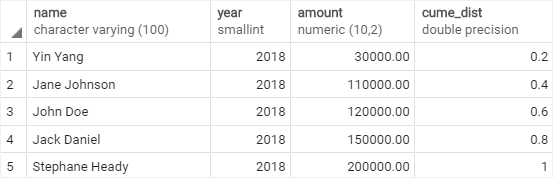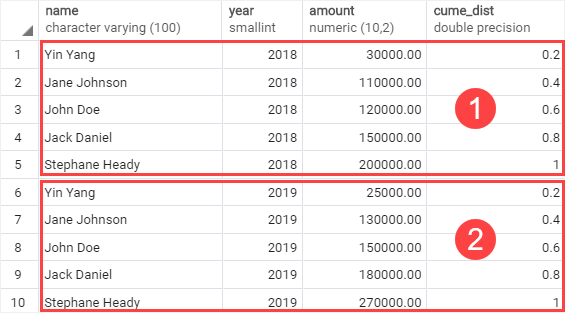# PostgreSQL Tutorial: CUME_DIST Function

August 7, 2023

Summary: in this tutorial, you will learn how to use the PostgreSQL `CUME_DIST()` function to calculate the cumulative distribution of a value within a set of values.

## PostgreSQL `CUME_DIST()` function overview

Sometimes, you may want to create a report that shows the top or bottom x% values from a data set, for example, top 1% of products by revenue. Fortunately, PostgreSQL provides us with the `CUME_DIST()` function to calculate it.

The `CUME_DIST()` function returns the cumulative distribution of a value within a set of values. In other words, it returns the relative position of a value in a set of values.

The syntax of the `CUME_DIST()` function is as follows:

`````` CUME_DIST() OVER (
[PARTITION BY partition_expression, ... ]
ORDER BY sort_expression [ASC | DESC], ...
)
``````

Let’s examine this syntax in detail.

### `PARTITION BY` clause

The `PARTITION BY` clause divides rows into multiple partitions to which the function is applied.

The `PARTITION BY` clause is optional. If you skip it, the `CUME_DIST()` function will treat the whole result set as a single partition.

### `ORDER BY` clause

The `ORDER BY` clause sorts rows in each partition to which the `CUME_DIST()` function is applied.

### Return value

The `CUME_DIST()` returns a double precision value which is greater than 0 and less than or equal to 1:

``````0 < CUME_DIST() <= 1
``````

The function returns the same cumulative distribution values for the same tie values.

## PostgreSQL `CUME_DIST()` examples

First, create a new table named `sales_stats` that stores the sales revenue by employees:

``````CREATE TABLE sales_stats(
name VARCHAR(100) NOT NULL,
year SMALLINT NOT NULL CHECK (year > 0),
amount DECIMAL(10,2) CHECK (amount >= 0),
PRIMARY KEY (name,year)
);
``````

Second, insert some rows into the `sales_stats` table:

``````INSERT INTO
sales_stats(name, year, amount)
VALUES
('John Doe',2018,120000),
('Jane Doe',2018,110000),
('Jack Daniel',2018,150000),
('Yin Yang',2018,30000),
('John Doe',2019,150000),
('Jane Doe',2019,130000),
('Jack Daniel',2019,180000),
('Yin Yang',2019,25000),
``````

The following examples help you get a better understanding of the `CUME_DIST()` function.

### 1) Using PostgreSQL `CUME_DIST()` function over a result set example

The following example returns the sales amount percentile for each sales employee in 2018:

``````SELECT
name,
year,
amount,
CUME_DIST() OVER (
ORDER BY amount
)
FROM
sales_stats
WHERE
year = 2018;
``````

Here is the output:As clearly shown in the output, we can find that 80% of sales employees have sales less than or equal to 150K in 2018.

### 2) Using PostgreSQL `CUME_DIST()` function over a partition example

The following example uses the `CUME_DIST()` function to calculate the sales percentile for each sales employee in 2018 and 2019.

``````SELECT
name,
year,
amount,
CUME_DIST() OVER (
PARTITION BY year
ORDER BY amount
)
FROM
sales_stats;
``````

Here is the output:In this example:

• The `PARTITION BY`clause divided the rows into two partitions by the year 2018 and 2019.
• The `ORDER BY` clause sorted sales amount of every employee in each partition from high to low to which the `CUME_DIST()` function is applied.

In this tutorial, you have learned how to use the PostgreSQL `CUME_DIST()` function to calculate the cumulative distribution of a value in a group of values.